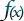# Tecplot 360 Basics: Data Alter

## Description

Change the values of entire variables or specific data points, or create new variables in Tecplot 360. This quick tutorial about Data Alter shows you how to do it. The video covers the Specify Equations Syntax in Tecplot 360. We will be calculating a commonly desired variable which is often not included in exported data sets: velocity magnitude.

Use Data>Alter>Specify Equations (or click thetoolbar button) to alter data in existing zones.In Tecplot equation syntax, creating a new variable or referencing a variable by name requires surrounding it in curly braces. Variables on the right side of equations may be referenced by two other methods:

The first method is referencing the variable by the variable index. The second method is referencing the variable assigned to the u and v vector component variables. In the second case Tecplot’s assigned variables axis or velocity components must be defined.

Common mathematical functions such as SIN, COS, SQRT are included in Tecplot equation syntax, in addition to other common mathematical operands. Most notably a double star (**) indicates squared or second power.

All three of these equations will achieve the same result for our velocity magnitude calculation:

• {Vmag} = sqrt({X Velocity}**2 + {Y Velocity}**2)
• {Vmag} = sqrt(V4**2 + V7**2)
• {Vmag} = sqrt(u**2 + v**2)